# 5 Data Wrangling via dplyr

Let’s briefly recap where we have been so far and where we are headed. In Chapter 4, we discussed what it means for data to be tidy. We saw that this refers to observations corresponding to rows and variables being stored in columns (one variable for every column). The entries in the data frame correspond to different combinations of observations (specific instances of observational units) and variables. In the flights data frame, we saw that each row corresponds to a different flight leaving New York City. In other words, the observational unit of the flights tidy data frame is a flight. The variables are listed as columns, and for flights these columns include both quantitative variables like dep_delay and distance and also categorical variables like carrier and origin. An entry in the table corresponds to a particular flight on a given day and a particular value of a given variable representing that flight.

Armed with this knowledge and looking back on Chapter 3, we see that organizing data in this tidy way makes it easy for us to produce graphics, specifically a set of 5 common graphics we termed the 5 Named Graphics (5NG):

1. scatterplots
2. linegraphs
3. boxplots
4. histograms
5. barplots

We can simply specify what variable/column we would like on one axis, (if applicable) what variable we’d like on the other axis, and what type of plot we’d like to make by specifying the geometric object in question. We can also vary aesthetic attributes of the geometric objects in question (points, lines, bar), such as the size and color, along the values of another variable in this tidy dataset. Recall the Gapminder example from Figure 3.1.

Lastly, in a few spots in Chapter 3 and Chapter 4, we hinted at some ways to summarize and wrangle data to suit your needs, using the filter() and inner_join() functions. This chapter expands on these functions by giving a variety of examples using what we term the Five Main Verbs (5MV) in the dplyr package (Wickham et al. 2017).

### Needed packages

Let’s load all the packages needed for this chapter (this assumes you’ve already installed them). If needed, read Section 2.3 for information on how to install and load R packages.

library(dplyr)
library(ggplot2)
library(nycflights13)
library(knitr)

## 5.1 The pipe %>%

Before we introduce the five main verbs, we first introduce the pipe operator (%>%). Just as the + sign was used to add layers to a plot created using ggplot(), the pipe operator allows us to chain together dplyr data wrangling functions. The pipe operator can be read as “then”. The %>% operator allows us to go from one step in dplyr to the next easily so we can, for example:

• filter our data frame to only focus on a few rows then
• group_by another variable to create groups then
• summarize this grouped data to calculate the mean for each level of the group.

The piping syntax will be our major focus throughout the rest of this book and you’ll find that you’ll quickly be addicted to the chaining with some practice.

## 5.2 Five Main Verbs - The 5MV

The d in dplyr stands for data frames, so the functions in dplyr are built for working with objects of the data frame type. For now, we focus on the 5MV: the five most commonly used functions that help wrangle and summarize data. A description of these verbs follows, with each subsection devoted to an example of that verb, or a combination of a few verbs, in action.

• filter: Pick rows based on conditions about their values
• summarize: Create summary measures of variables either
• over the entire data frame or
• over groups of observations on variables using group_by
• mutate: Create a new variable in the data frame by mutating existing ones
• arrange: Arrange/sort the rows based on one or more variables

Just as we had the Five Named Graphs for data visualization using ggplot2 in Chapter 3, we have the 5MV here (The Five Main Verbs in dplyr) for data wrangling. All of the 5MVs follow the same syntax, with the argument before the pipe %>% being the name of the data frame, then the name of the verb, followed with other arguments specifying which criteria you’d like the verb to work with in parentheses.

Keep in mind, there are more advanced functions than just these five and you’ll see some examples of this near the end of this chapter in 5.4, but with the 5MV you’ll be able to perform a broad array of data wrangling tasks.

### 5.2.1 5MV#1: Filter observations using filter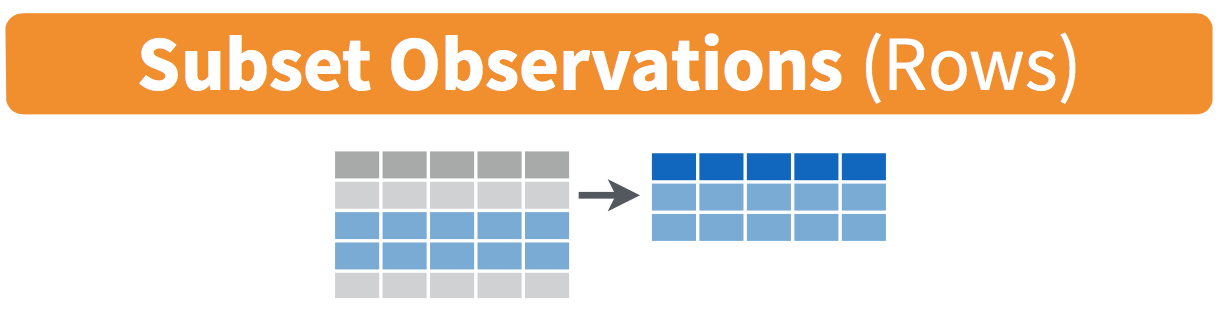Figure 5.1: Filter diagram from Data Wrangling with dplyr and tidyr cheatsheet

The filter function here works much like the “Filter” option in Microsoft Excel; it allows you to specify criteria about values of a variable in your dataset and then chooses only those rows that match that criteria. We begin by focusing only on flights from New York City to Portland, Oregon. The dest code (or airport code) for Portland, Oregon is "PDX". Run the following and look at the resulting spreadsheet to ensure that only flights heading to Portland are chosen here:

portland_flights <- flights %>%
filter(dest == "PDX")
View(portland_flights)

Note the following:

• The ordering of the commands:
• Take the data frame flights then
• filter the data frame so that only those where the dest equals "PDX" are included.
• The double equal sign == for testing for equality, and not =. You are almost guaranteed to make the mistake at least once of only including one equals sign.

You can combine multiple criteria together using operators that make comparisons:

• | corresponds to “or”
• & corresponds to “and”

We can often skip the use of & and just separate our conditions with a comma. You’ll see this in the example below.

In addition, you can use other mathematical checks (similar to ==):

• > corresponds to “greater than”
• < corresponds to “less than”
• >= corresponds to “greater than or equal to”
• <= corresponds to “less than or equal to”
• != corresponds to “not equal to”

To see many of these in action, let’s select all flights that left JFK airport heading to Burlington, Vermont ("BTV") or Seattle, Washington ("SEA") in the months of October, November, or December. Run the following

btv_sea_flights_fall <- flights %>%
filter(origin == "JFK", (dest == "BTV" | dest == "SEA"), month >= 10)
View(btv_sea_flights_fall)

Note: even though colloquially speaking one might say “all flights leaving Burlington, Vermont and Seattle, Washington,” in terms of computer logical operations, we really mean “all flights leaving Burlington, Vermont or Seattle, Washington.” For a given row in the data, dest can be “BTV”, “SEA”, or something else, but not “BTV” and “SEA” at the same time.

Another example uses the ! to pick rows that don’t match a condition. The ! can be read as “not”. Here we are selecting rows corresponding to flights that didn’t go to Burlington, VT or Seattle, WA.

not_BTV_SEA <- flights %>%
filter(!(dest == "BTV" | dest == "SEA"))
View(not_BTV_SEA)

As a final note we point out that filter() should often be the first verb you’ll apply to your data. This cleans your dataset to only those rows you care about, or put differently, it narrows down the scope to just the observations your care about.

Learning check

(LC5.1) What’s another way using the “not” operator ! we could filter only the rows that are not going to Burlington, VT nor Seattle, WA in the flights data frame? Test this out using the code above.

### 5.2.2 5MV#2: Summarize variables using summarize

The next common task when working with data is to be able to summarize data: take a large number of values and summarize then with a single value. While this may seem like a very abstract idea, something has simple as the sum, the smallest value, and the largest values are all summaries of a large number of values.Figure 5.2: Summarize diagram from Data Wrangling with dplyr and tidyr cheatsheet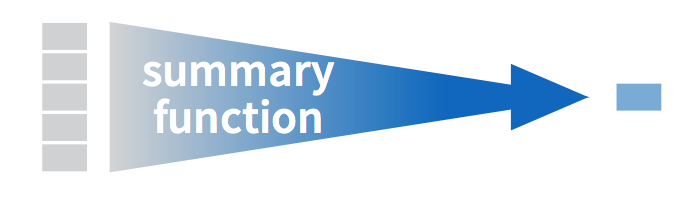Figure 5.3: Another summarize diagram from Data Wrangling with dplyr and tidyr cheatsheet

We can calculate the standard deviation and mean of the temperature variable temp in the weather data frame of nycflights13 in one step using the summarize (or equivalently using the UK spelling summarise) function in dplyr (See Appendix A):

summary_temp <- weather %>%
summarize(mean = mean(temp), std_dev = sd(temp))
kable(summary_temp)
mean std_dev
NA NA

We’ve created a small data frame here called summary_temp that includes both the mean and the std_dev of the temp variable in weather. Notice as shown in Figures 5.2 and 5.3, the data frame weather went from many rows to a single row of just the summary values in the data frame summary_temp.

But why are the values returned NA? This stands for “not available or not applicable” and is how R encodes missing values; if in a data frame for a particular row and column no value exists, NA is stored instead. Furthermore, by default any time you try to summarize a number of values (using mean() and sd() for example) that has one or more missing values, then NA is returned.

Values can missing missing for many reasons. Perhaps the data was collected but someone forgot to enter it? Perhaps the data was not collected at all because it was too difficult? Perhaps there was an erroneous value that someone entered that has been correct to read as missing? You’ll often encounter issues with missing values.

You can summarize all non-missing values by setting the na.rm argument to TRUE (rm is short for “remove”). This will remove any NA missing values and only return the summary value for all non-missing values. So the code below computes the mean and standard deviation of all non-missing values. Notice how the na.rm=TRUE are set as arguments to the mean() and sd() functions, and not to the summarize() function.

summary_temp <- weather %>%
summarize(mean = mean(temp, na.rm = TRUE), std_dev = sd(temp, na.rm = TRUE))
kable(summary_temp)
mean std_dev
55.2 17.78

It is not good practice to include a na.rm = TRUE in your summary commands by default; you should attempt to run them without this argument. This is because removing missing data can have an impact on your analyses. In fact, an entire branch of the field of statistics deals with missing data. The take away point is that na.rm = TRUE should only be used after you aware of the implications of its use (see the Learning Checks below for an example).

What other summary functions can we use inside the summarize() verb? Any function in R that takes a vector of values and returns just one. Here are just a few:

• min() and max(): the minimum and maximum values respectively
• IQR(): Interquartile range
• sum(): the sum
• n(): a count of the number of rows/observations in each group. This particular summary function will make more sense when group_by() is covered in Subsection 5.2.3.

Learning check

(LC5.2) Say a doctor is studying the effect of smoking on lung cancer for a large number of patients who have records measured at five year intervals. She notices that a large number of patients have missing data points because the patient has died, so she chooses to ignore these patients in his analysis. What is wrong with this doctor’s approach?

(LC5.3) Modify the above summarize function to create summary_temp to also use the n() summary function: summarize(count = n()). What does the returned value correspond to?

(LC5.4) Why doesn’t the following code work? Run the code line by line instead of all at once, and then look at the data. In other words, run summary_temp <- weather %>% summarize(mean = mean(temp, na.rm = TRUE)) first.

summary_temp <- weather %>%
summarize(mean = mean(temp, na.rm = TRUE)) %>%
summarize(std_dev = sd(temp, na.rm = TRUE))

### 5.2.3 5MV#3: Group rows using group_by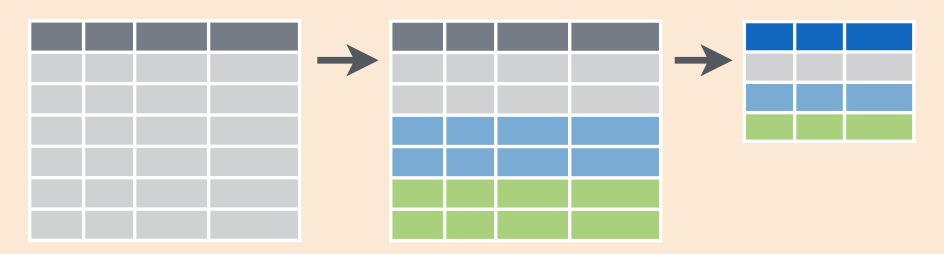Figure 5.4: Group by and summarize diagram from Data Wrangling with dplyr and tidyr cheatsheet

It’s often more useful to summarize a variable based on the groupings of another variable. Let’s say, we are interested in the mean and standard deviation of temperatures but grouped by month. To be more specific: we want the mean and standard deviation of temperatures

1. split by month.
2. sliced by month.
3. aggregated by month.
4. collapsed over month.

Run the following code (recall the discussion of the kable() function from the knitr package in Subsection 2.4.3):

summary_monthly_temp <- weather %>%
group_by(month) %>%
summarize(mean = mean(temp, na.rm = TRUE),
std_dev = sd(temp, na.rm = TRUE))
kable(summary_monthly_temp)
month mean std_dev
1 35.64 10.185
2 34.15 6.940
3 39.81 6.225
4 51.67 8.785
5 61.59 9.609
6 72.14 7.603
7 80.01 7.148
8 74.40 5.171
9 67.43 8.476
10 60.03 8.830
11 45.11 10.502
12 38.37 9.941

This code is identical to the previous code that created summary_temp, with an extra group_by(month) added. Grouping the weather dataset by month and then passing this new data frame into summarize yields a data frame that shows the mean and standard deviation of temperature for each month in New York City. Note: Since each row in summary_monthly_temp represents a summary of different rows in weather, the observational units have changed.

It is important to note that group_by doesn’t change the data frame. It sets meta-data (data about the data), specifically the group structure of the data. It is only after we apply the summarize function that the data frame changes.

If we would like to remove this group structure meta-data, we can pipe the resulting data frame into the ungroup() function. For example, say the group structure meta-data is set to be by month via group_by(month), all future summarizations will be reported on a month-by-month basis. If however, we would like to no longer have this and have all summarizations be for all data in a single group (in this case over the entire year of 2013), then pipe the data frame in question through and ungroup() to remove this.

We now revisit the n() counting summary function we introduced in the previous section. For example, suppose we’d like to get a sense for how many flights departed each of the three airports in New York City:

by_origin <- flights %>%
group_by(origin) %>%
summarize(count = n())
kable(by_origin)
origin count
EWR 120835
JFK 111279
LGA 104662

We see that Newark ("EWR") had the most flights departing in 2013 followed by "JFK" and lastly by LaGuardia ("LGA"). Note there is a subtle but important difference between sum() and n(). While sum() simply adds up a large set of numbers, the latter counts the number of times each of many different values occur.

You are not limited to grouping by one variable! Say you wanted to know the number of flights leaving each of the three New York City airports for each month, we can also group by a second variable month: group_by(origin, month).

by_monthly_origin <- flights %>%
group_by(origin, month) %>%
summarize(count = n())
kable(by_monthly_origin)
origin month count
EWR 1 9893
EWR 2 9107
EWR 3 10420
EWR 4 10531
EWR 5 10592
EWR 6 10175
EWR 7 10475
EWR 8 10359
EWR 9 9550
EWR 10 10104
EWR 11 9707
EWR 12 9922
JFK 1 9161
JFK 2 8421
JFK 3 9697
JFK 4 9218
JFK 5 9397
JFK 6 9472
JFK 7 10023
JFK 8 9983
JFK 9 8908
JFK 10 9143
JFK 11 8710
JFK 12 9146
LGA 1 7950
LGA 2 7423
LGA 3 8717
LGA 4 8581
LGA 5 8807
LGA 6 8596
LGA 7 8927
LGA 8 8985
LGA 9 9116
LGA 10 9642
LGA 11 8851
LGA 12 9067

Alternatively, you can use the shortcut count() function in dplyr to get the same result:

by_monthly_origin2 <- flights %>%
dplyr::count(origin, month)
kable(by_monthly_origin2)
origin month n
EWR 1 9893
EWR 2 9107
EWR 3 10420
EWR 4 10531
EWR 5 10592
EWR 6 10175
EWR 7 10475
EWR 8 10359
EWR 9 9550
EWR 10 10104
EWR 11 9707
EWR 12 9922
JFK 1 9161
JFK 2 8421
JFK 3 9697
JFK 4 9218
JFK 5 9397
JFK 6 9472
JFK 7 10023
JFK 8 9983
JFK 9 8908
JFK 10 9143
JFK 11 8710
JFK 12 9146
LGA 1 7950
LGA 2 7423
LGA 3 8717
LGA 4 8581
LGA 5 8807
LGA 6 8596
LGA 7 8927
LGA 8 8985
LGA 9 9116
LGA 10 9642
LGA 11 8851
LGA 12 9067

Learning check

(LC5.5) Recall from Chapter 3 when we looked at plots of temperatures by months in NYC. What does the standard deviation column in the summary_monthly_temp data frame tell us about temperatures in New York City throughout the year?

(LC5.6) What code would be required to get the mean and standard deviation temperature for each day in 2013 for NYC?

(LC5.7) Recreate by_monthly_origin, but instead of grouping via group_by(origin, month), group variables in a different order group_by(month, origin). What differs in the resulting dataset?

(LC5.8) How could we identify how many flights left each of the three airports for each carrier?

(LC5.9) How does the filter operation differ from a group_by followed by a summarize?

### 5.2.4 5MV#4: Create new variables/change old variables using mutate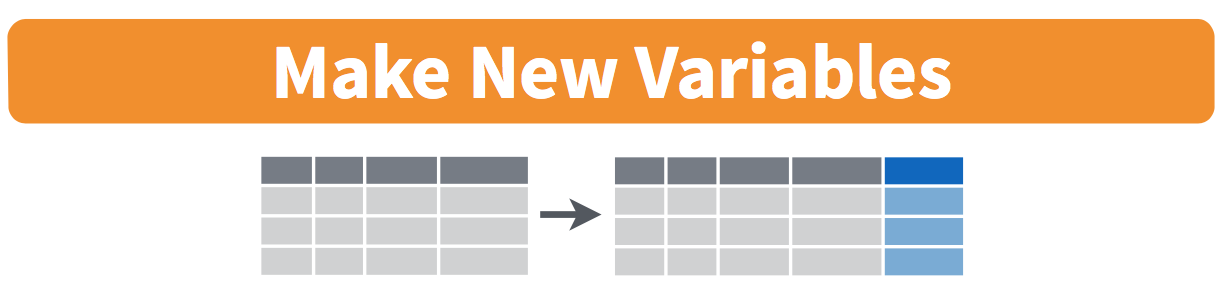Figure 5.5: Mutate diagram from Data Wrangling with dplyr and tidyr cheatsheet

When looking at the flights dataset, there are some clear additional variables that could be calculated based on the values of variables already in the dataset. Passengers are often frustrated when their flights departs late, but change their mood a bit if pilots can make up some time during the flight to get them to their destination close to when they expected to land. This is commonly referred to as “gain” and we will create this variable using the mutate function. Note that we have also overwritten the flights data frame with what it was before as well as an additional variable gain here, or put differently, the mutate() command outputs a new data frame which then gets saved over the original flights data frame.

flights <- flights %>%
mutate(gain = dep_delay - arr_delay)

Why did we overwrite flights instead of assigning the resulting data frame to a new object, like flights_with_gain? As a rough rule of thumb, as long as you are not losing information that you might need later, it’s acceptable practice to overwrite data frames. However, if you overwrite existing variables and/or change the observational units, recovering the original information might prove difficult. In this case, it might make sense to create a new data object.

Let’s look at summary measures of this gain variable and even plot it in the form of a histogram:

gain_summary <- flights %>%
summarize(
min = min(gain, na.rm = TRUE),
q1 = quantile(gain, 0.25, na.rm = TRUE),
median = quantile(gain, 0.5, na.rm = TRUE),
q3 = quantile(gain, 0.75, na.rm = TRUE),
max = max(gain, na.rm = TRUE),
mean = mean(gain, na.rm = TRUE),
sd = sd(gain, na.rm = TRUE),
missing = sum(is.na(gain))
)
kable(gain_summary)
min q1 median q3 max mean sd missing
-196 -3 7 17 109 5.66 18.04 9430

We’ve recreated the summary function we saw in Chapter 3 here using the summarize function in dplyr.

ggplot(data = flights, mapping = aes(x = gain)) +
geom_histogram(color = "white", bins = 20)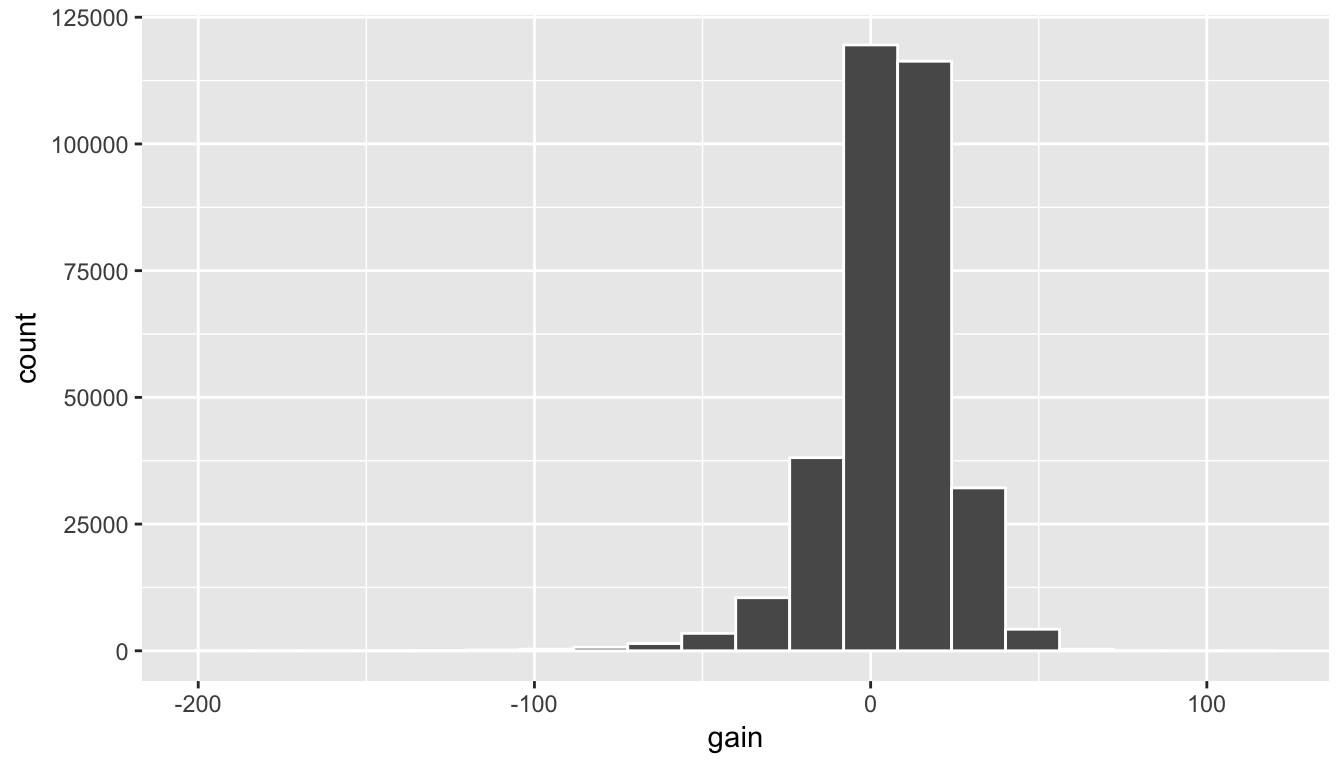Figure 5.6: Histogram of gain variable

We can also create multiple columns at once and even refer to columns that were just created in a new column. Hadley and Garrett produce one such example in Chapter 5 of “R for Data Science” (Grolemund and Wickham 2016):

flights <- flights %>%
mutate(
gain = dep_delay - arr_delay,
hours = air_time / 60,
gain_per_hour = gain / hours
)

Learning check

(LC5.10) What do positive values of the gain variable in flights correspond to? What about negative values? And what about a zero value?

(LC5.11) Could we create the dep_delay and arr_delay columns by simply subtracting dep_time from sched_dep_time and similarly for arrivals? Try the code out and explain any differences between the result and what actually appears in flights.

(LC5.12) What can we say about the distribution of gain? Describe it in a few sentences using the plot and the gain_summary data frame values.

### 5.2.5 5MV#5: Reorder the data frame using arrange

One of the most common things people working with data would like to do is sort the data frames by a specific variable in a column. Have you ever been asked to calculate a median by hand? This requires you to put the data in order from smallest to highest in value. The dplyr package has a function called arrange that we will use to sort/reorder our data according to the values of the specified variable. This is often used after we have used the group_by and summarize functions as we will see.

Let’s suppose we were interested in determining the most frequent destination airports from New York City in 2013:

freq_dest <- flights %>%
group_by(dest) %>%
summarize(num_flights = n())
freq_dest
# A tibble: 105 x 2
dest num_flights
<chr>       <int>
1   ABQ         254
2   ACK         265
3   ALB         439
4   ANC           8
5   ATL       17215
6   AUS        2439
7   AVL         275
8   BDL         443
9   BGR         375
10   BHM         297
# ... with 95 more rows

You’ll see that by default the values of dest are displayed in alphabetical order here. We are interested in finding those airports that appear most:

freq_dest %>% arrange(num_flights)
# A tibble: 105 x 2
dest num_flights
<chr>       <int>
1   LEX           1
2   LGA           1
3   ANC           8
4   SBN          10
5   HDN          15
6   MTJ          15
7   EYW          17
8   PSP          19
9   JAC          25
10   BZN          36
# ... with 95 more rows

This is actually giving us the opposite of what we are looking for. It tells us the least frequent destination airports first. To switch the ordering to be descending instead of ascending we use the desc (descending) function:

freq_dest %>% arrange(desc(num_flights))
# A tibble: 105 x 2
dest num_flights
<chr>       <int>
1   ORD       17283
2   ATL       17215
3   LAX       16174
4   BOS       15508
5   MCO       14082
6   CLT       14064
7   SFO       13331
8   FLL       12055
9   MIA       11728
10   DCA        9705
# ... with 95 more rows

## 5.3 Joining data frames

Another common task is joining/merging two different datasets. For example, in the flights data, the variable carrier lists the carrier code for the different flights. While "UA" and "AA" might be somewhat easy to guess for some (United and American Airlines), what are “VX”, “HA”, and “B6”? This information is provided in a separate data frame airlines.

View(airlines)

We see that in airports, carrier is the carrier code while name is the full name of the airline. Using this table, we can see that “VX”, “HA”, and “B6” correspond to Virgin America, Hawaiian Airlines, and JetBlue respectively. However, will we have to continually look up the carrier’s name for each flight in the airlines dataset? No! Instead of having to do this manually, we can have R automatically do the “looking up” for us.

Note that the values in the variable carrier in flights match the values in the variable carrier in airlines. In this case, we can use the variable carrier as a key variable to join/merge/match the two data frames by. Hadley and Garrett (Grolemund and Wickham 2016) created the following diagram to help us understand how the different datasets are linked: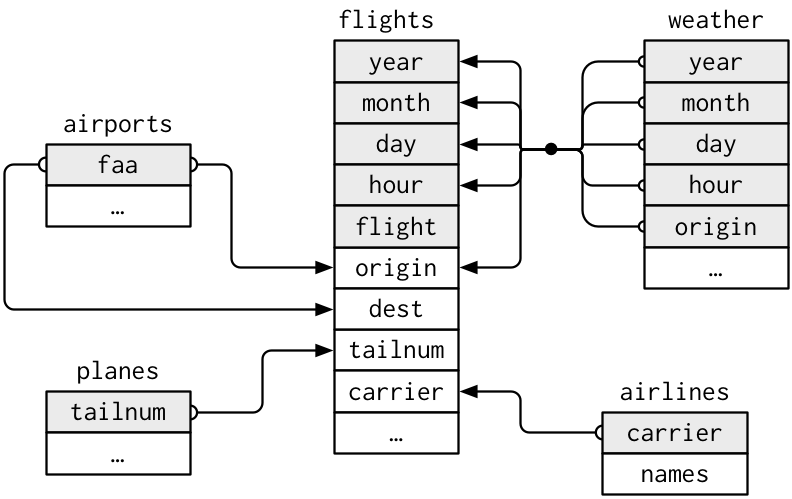Figure 5.7: Data relationships in nycflights13 from R for Data Science

### 5.3.1 Joining by key variables

In both flights and airlines, the key variable we want to join/merge/match the two data frames with has the same name in both datasets: carriers. We make use of the inner_join() function to join by the variable carrier.

flights_joined <- flights %>%
inner_join(airlines, by = "carrier")
View(flights)
View(flights_joined)

We observed that the flights and flights_joined are identical except that flights_joined has an additional variable name whose values were drawn from airlines.

A visual representation of the inner_join is given below (Grolemund and Wickham 2016):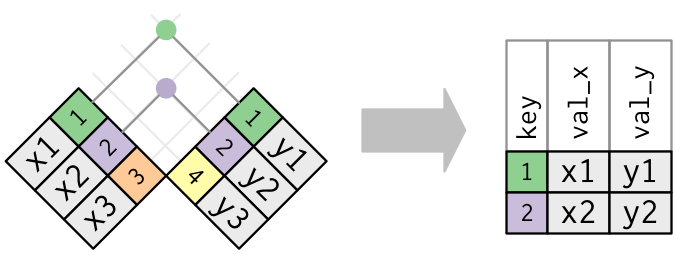Figure 5.8: Diagram of inner join from R for Data Science

There are more complex joins available, but the inner_join will solve nearly all of the problems you’ll face in our experience.

### 5.3.2 Joining by key variables with different names

Say instead, you are interested in all the destinations of flights from NYC in 2013 and ask yourself:

• “What cities are these airports in?”
• “Is "ORD" Orlando?”
• “Where is "FLL"?

The airports data frame contains airport codes:

View(airports)

However, looking at both the airports and flights and the visual representation of the relations between the data frames in Figure 5.8, we see that in:

• airports the airport code is in the variable faa
• flights the airport code is in the variable origin

So to join these two datasets, our inner_join operation involves a by argument that accounts for the different names:

flights %>%
inner_join(airports, by = c("dest" = "faa"))

Let’s construct the sequence of commands that computes the number of flights from NYC to each destination, but also includes information about each destination airport:

named_dests <- flights %>%
group_by(dest) %>%
summarize(num_flights = n()) %>%
arrange(desc(num_flights)) %>%
inner_join(airports, by = c("dest" = "faa")) %>%
rename(airport_name = name)
View(named_dests)

In case you didn’t know, "ORD" is the airport code of Chicago O’Hare airport and "FLL" is the main airport in Fort Lauderdale, Florida, which we can now see in our named_freq_dests data frame.

Learning check

(LC5.13) Looking at Figure 5.7, when joining flights and weather (or, in other words, matching the hourly weather values with each flight), why do we need to join by all of year, month, day, hour, and origin, and not just hour?

(LC5.14) What surprises you about the top 10 destinations from NYC in 2013?

## 5.4 Optional: Other verbs

On top of the following examples of other verbs, if you’d like to see more examples on using dplyr, the 5MV, and %>% with the nycflights13 dataset, check out Chapter 5 of Hadley and Garrett’s book (Grolemund and Wickham 2016).

### 5.4.1 Select variables using selectFigure 5.9: Select diagram from Data Wrangling with dplyr and tidyr cheatsheet

We’ve seen that the flights data frame in the nycflights13 package contains many different variables. The names function gives a listing of all the columns in a data frame; in our case you would run names(flights). You can also identify these variables by running the glimpse function in the dplyr package:

glimpse(flights)

However, say you only want to consider two of these variables, say carrier and flight. You can select these:

flights %>%
select(carrier, flight)

Another one of these variables is year. If you remember the original description of the flights data frame (or by running ?flights), you’ll remember that this data correspond to flights in 2013 departing New York City. The year variable isn’t really a variable here in that it doesn’t vary… flights actually comes from a larger dataset that covers many years. We may want to remove the year variable from our dataset since it won’t be helpful for analysis in this case. We can deselect year by using the - sign:

flights_no_year <- flights %>%
select(-year)
names(flights_no_year)

Or we could specify a ranges of columns:

flight_arr_times <- flights %>%
select(month:day, arr_time:sched_arr_time)
flight_arr_times

The select function can also be used to reorder columns in combination with the everything helper function. Let’s suppose we’d like the hour, minute, and time_hour variables, which appear at the end of the flights dataset, to actually appear immediately after the day variable:

flights_reorder <- flights %>%
select(month:day, hour:time_hour, everything())
names(flights_reorder)

in this case everything() picks up all remaining variables. Lastly, the helper functions starts_with, ends_with, and contains can be used to choose column names that match those conditions:

flights_begin_a <- flights %>%
select(starts_with("a"))
flights_begin_a
flights_delays <- flights %>%
select(ends_with("delay"))
flights_delays
flights_time <- flights %>%
select(contains("time"))
flights_time

### 5.4.2 Rename variables using rename

Another useful function is rename, which as you may suspect renames one column to another name. Suppose we wanted dep_time and arr_time to be departure_time and arrival_time instead in the flights_time data frame:

flights_time_new <- flights %>%
select(contains("time")) %>%
rename(departure_time = dep_time,
arrival_time = arr_time)
names(flights_time)

Note that in this case we used a single = sign with the rename(). Ex: departure_time = dep_time. This is because we are not testing for equality like we would using ==, but instead we want to assign a new variable departure_time to have the same values as dep_time and then delete the variable dep_time.

It’s easy to forget if the new name comes before or after the equals sign. I usually remember this as “New Before, Old After” or NBOA. You’ll receive an error if you try to do it the other way:

Error: Unknown variables: departure_time, arrival_time.

### 5.4.3 Find the top number of values using top_n

We can also use the top_n function which automatically tells us the most frequent num_flights. We specify the top 10 airports here:

named_dests %>%
top_n(n = 10, wt = num_flights)

We’ll still need to arrange this by num_flights though:

named_dests  %>%
top_n(n = 10, wt = num_flights) %>%
arrange(desc(num_flights))

Note: Remember that I didn’t pull the n and wt arguments out of thin air. They can be found by using the ? function on top_n.

We can go one stop further and tie together the group_by and summarize functions we used to find the most frequent flights:

ten_freq_dests <- flights %>%
group_by(dest) %>%
summarize(num_flights = n()) %>%
arrange(desc(num_flights)) %>%
top_n(n = 10)
View(ten_freq_dests)

Learning check

(LC5.15) What are some ways to select all three of the dest, air_time, and distance variables from flights? Give the code showing how to do this in at least three different ways.

(LC5.16) How could one use starts_with, ends_with, and contains to select columns from the flights data frame? Provide three different examples in total: one for starts_with, one for ends_with, and one for contains.

(LC5.17) Why might we want to use the select function on a data frame?

(LC5.18) Create a new data frame that shows the top 5 airports with the largest arrival delays from NYC in 2013.

## 5.5 Conclusion

### 5.5.1 Review questions

Review questions have been designed using the fivethirtyeight R package (Ismay and Chunn 2017) with links to the corresponding FiveThirtyEight.com articles in our free DataCamp course Effective Data Storytelling using the tidyverse. The material in this chapter is covered in the chapters of the DataCamp course available below:

### 5.5.2 What’s to come?

We will see in Chapter 6 many of the same ideas we have seen with hypothesis testing and confidence intervals in the last two chapters. Regression is frequently associated both correctly and incorrectly with statistics and data analysis, so you’ll need to make sure you understand when it is appropriate and when it is not.

### 5.5.3 Resources

As we saw with the RStudio cheatsheet on data visualization, RStudio has also created a cheatsheet for data wrangling entitled “Data Transformation with dplyr”.

### 5.5.4 Script of R code

An R script file of all R code used in this chapter is available here.